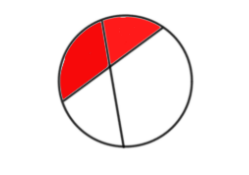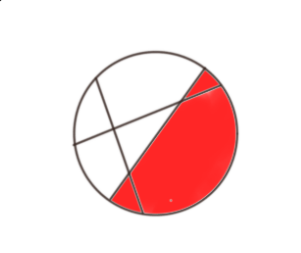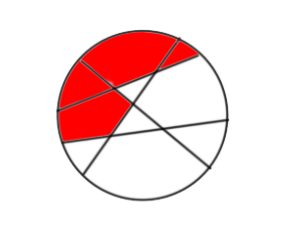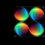# Dividing a Circle into the Maximum Number of Regions with n lines

Recently, I came across a question that required to find the maximum number of regions a circle can be divided into with 4 lines, considering regions fromed inside the circle only. The question was an easy one; a fair bit of trial-and-error popped out the answer as $11$. But what if there were, let's say, $100$ lines? Obviously, trial-and-error here would drain the soul out of me! So, I started investigating the mechanism that created the maximum number of regions for $n$ lines, and derived a formula which would give us the answer directly.

First of all, I found that when I was trying for the $n=4$ case, what I was essentialy trying to do is to make sure that every new line I drew cut all the previous lines, thereby forming the maximum regions. To put it in another way, I sought to draw the $n^{th}$ line such that it cut all the previous $n-1$ lines.

After this realization, I tried to find out the number of regions that were being added after each new line was drawn. For example, when the first line is drawn, $2$ regions are formed.

When the second line is drawn, $2$ more regions are added to the figure. The regions shaded red are the newly added regions.When the third line is drawn cutting the previous $2$ lines, $3$ more regions are added.When the fourth line is drawn cutting the previous $3$ lines, $4$ more regions are formed.Thus, we have now found out a pattern.

Generalizing, the addition of the $n^{th}$ line results into the formation of $n$ new regions. This is because when you draw the $n^{th}$ line cuting the previous $n-1$ lines, you add a total of $n-1+1 = n$ regions.

This pattern can now be condensed into an Arithmetic Progression whose $n^{th}$ term represents the number of regions that are being added as each new line is drawn.

 1st 2nd 3rd 4th 5th nth 2 2 3 4 5 n

Note that all terms except the first one are a part of this AP with common difference $1$, so we add it separately. The total total number of terms in this AP are $n-1$. The first term is $2$. Apply the formula for the sum of an AP.

Maximum regions formed:

$2 + \dfrac{(n-1)}{2}\times [4+ (n-2)\times 1]$

= $2 + \dfrac{(n-1)}{2}(n+2)$

= $\dfrac{4 + n^2 + n - 2}{2}$

= $\boxed{\dfrac{n^2 + n + 2 }{2}}$Note by Anuj Shikarkhane
2 years, 7 months ago

This discussion board is a place to discuss our Daily Challenges and the math and science related to those challenges. Explanations are more than just a solution — they should explain the steps and thinking strategies that you used to obtain the solution. Comments should further the discussion of math and science.

When posting on Brilliant:

• Use the emojis to react to an explanation, whether you're congratulating a job well done , or just really confused .
• Ask specific questions about the challenge or the steps in somebody's explanation. Well-posed questions can add a lot to the discussion, but posting "I don't understand!" doesn't help anyone.
• Try to contribute something new to the discussion, whether it is an extension, generalization or other idea related to the challenge.

MarkdownAppears as
*italics* or _italics_ italics
**bold** or __bold__ bold
- bulleted- list
• bulleted
• list
1. numbered2. list
1. numbered
2. list
Note: you must add a full line of space before and after lists for them to show up correctly
paragraph 1paragraph 2

paragraph 1

paragraph 2

[example link](https://brilliant.org)example link
> This is a quote
This is a quote
    # I indented these lines
# 4 spaces, and now they show
# up as a code block.

print "hello world"
# I indented these lines
# 4 spaces, and now they show
# up as a code block.

print "hello world"
MathAppears as
Remember to wrap math in $$ ... $$ or $ ... $ to ensure proper formatting.
2 \times 3 $2 \times 3$
2^{34} $2^{34}$
a_{i-1} $a_{i-1}$
\frac{2}{3} $\frac{2}{3}$
\sqrt{2} $\sqrt{2}$
\sum_{i=1}^3 $\sum_{i=1}^3$
\sin \theta $\sin \theta$
\boxed{123} $\boxed{123}$

Sort by:

I think your table had a slight error. 1st line adds 1 region. (There is one region inside a circle with no lines, the first line splits this in two, so one is added)

Also, your 3rd picture makes no sense.

Nice formula, though.

- 2 years, 6 months ago